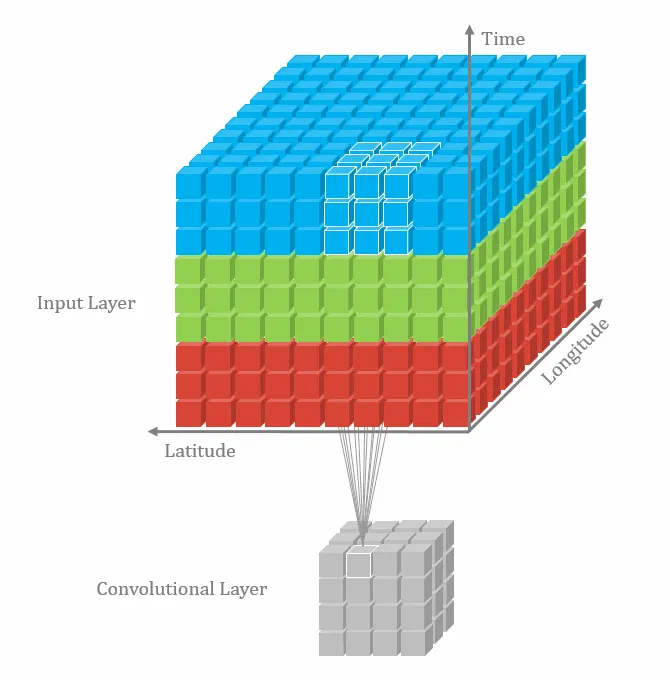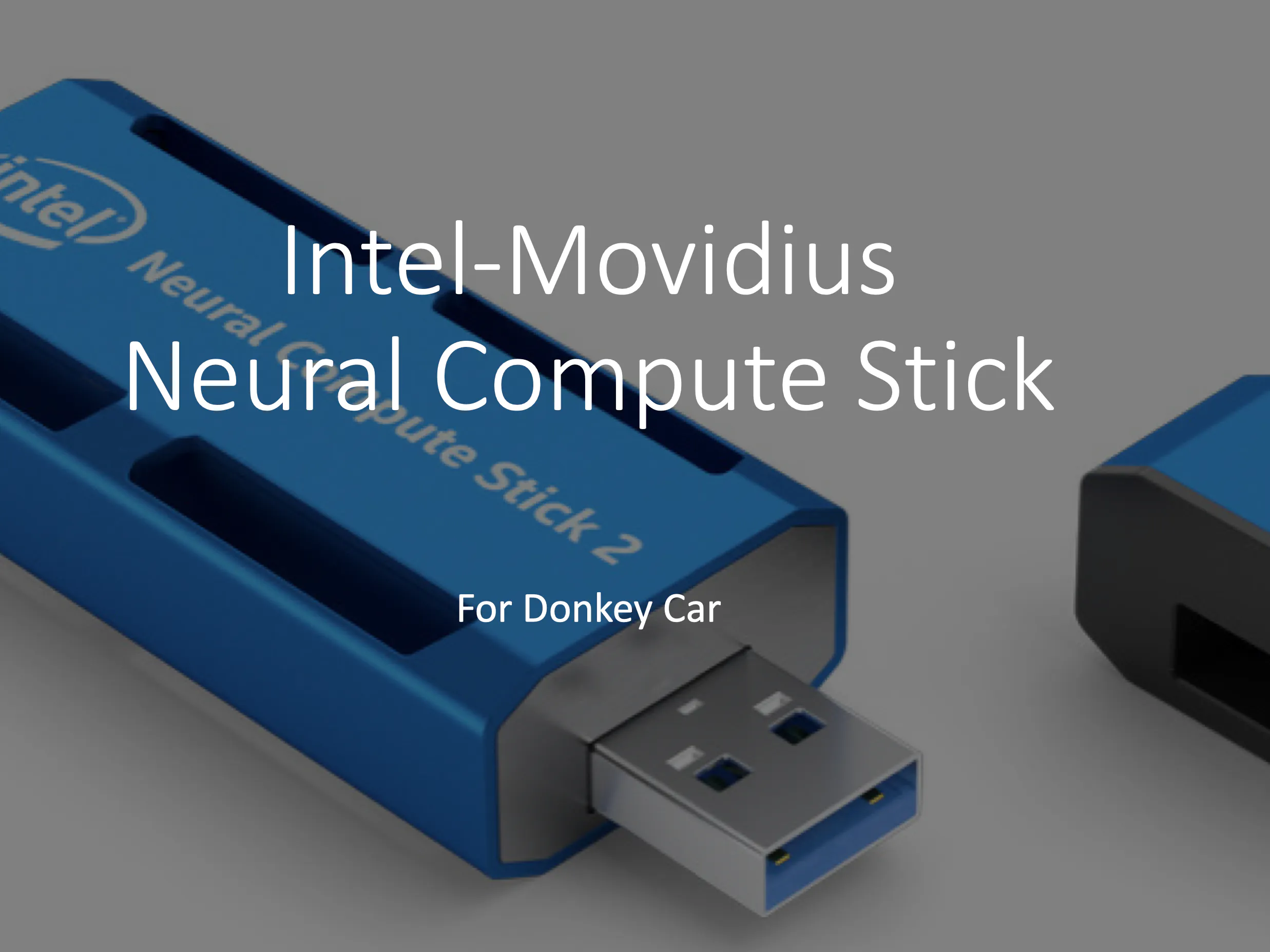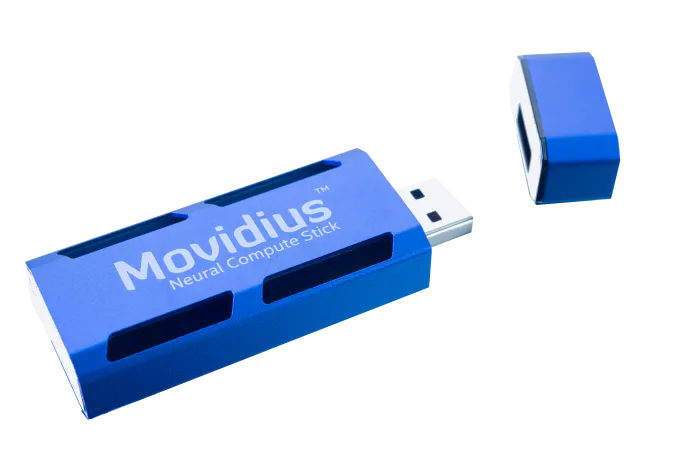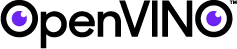Published

# Applying VPU to Improve Autonomous Donkey Car Performance

IntermediateProtip534## Things used in this project

### Hardware componentsIntel Movidius Neural Compute Stick
×1

### Software apps and online servicesIntel OpenVINO toolkit

## Code

### manage3dcanny.py

Python
```#!/usr/bin/env python3
"""
Scripts to drive a donkey 2 car and train a model for it.

Usage:
manage.py (drive) [--model=<model>] [--js] [--chaos]
manage.py (train) [--tub=<tub1,tub2,..tubn>]  (--model=<model>) [--base_model=<base_model>] [--no_cache]

Options:
-h --help        Show this screen.
--tub TUBPATHS   List of paths to tubs. Comma separated. Use quotes to use wildcards. ie "~/tubs/*"
--chaos          Add periodic random steering when manually driving
"""
import os
os.environ['KERAS_BACKEND'] = 'tensorflow'

from docopt import docopt
import donkeycar as dk

import numpy as np
from donkeycar.parts.camera import PiCamera
from donkeycar.parts.transform import Lambda
from donkeycar.parts.keras import KerasLinear
#from alg.cnn3d import KerasLinear
from donkeycar.parts.actuator import PCA9685, PWMSteering, PWMThrottle
from donkeycar.parts.datastore import TubGroup, TubWriter
from donkeycar.parts.web_controller import LocalWebController
from donkeycar.parts.clock import Timestamp
from donkeycar.parts.datastore import TubGroup, TubWriter
from donkeycar.parts.keras import KerasLinear
from donkeycar.parts.transform import Lambda
import cv2
import numpy as np
from armv7l.openvino.inference_engine import IENetwork,IEPlugin

# build a lookup table mapping the pixel values [0, 255] to
invGamma = 1.0 / gamma
table = np.array([((i / 255.0) ** invGamma) * 255
for i in np.arange(0, 256)]).astype("uint8")

# apply gamma correction using the lookup table
return cv2.LUT(image, table)

def region_of_interest(img):
# Define a blank matrix that matches the image height/width.
# Retrieve the number of color channels of the image.
channel_count = img.shape
# Create a match color with the same color channel counts.

# Fill inside the polygon
# Returning the image only where mask pixels match

def region_to_crop(img):

#print('here in crop')
y = 80
x = 0
h = 240
w = 320
crop_img = img[y:h, x:w]
return crop_img

def region_of_interest_proc(img):
# Define a blank matrix that matches the image height/width.
# Retrieve the number of color channels of the image.
# channel_count = img.shape
# Create a match color with the same color channel counts.

# Fill inside the polygon
# Returning the image only where mask pixels match

def lineDetection(srcImage):
srcImage = cv2.cvtColor(srcImage, cv2.COLOR_BGR2RGB)
srcImage = region_of_interest(srcImage)
srcImage = cv2.Canny(srcImage, 100, 200)
srcImage = region_to_crop(srcImage)
return srcImage

def drive(cfg, model_path=None, use_chaos=False):

"""
Construct a working robotic vehicle from many parts.
Each part runs as a job in the Vehicle loop, calling either
All parts are updated one after another at the framerate given in
cfg.DRIVE_LOOP_HZ assuming each part finishes processing in a timely manner.
Parts may have named outputs and inputs. The framework handles passing named outputs
to parts requesting the same named input.
"""
#Part to save multiple image arrays from camera
class NCS:
def __init__(self):
#hardware
#plugin = IEPlugin(device=device, plugin_dirs=plugin_dir)
#model
net = IENetwork.from_ir(model="/home/pi/mycar/ncs/3dcnn/tf_model.xml",weights="/home/pi/mycar/ncs/3dcnn/tf_model.bin")

def run(self, image):
res = exec_net.infer(inputs={input_blob: processedImg})
print(res)
return res

V = dk.vehicle.Vehicle()

clock = Timestamp()

cam = PiCamera(resolution=cfg.CAMERA_RESOLUTION)

ctr = LocalWebController(use_chaos=use_chaos)
inputs=['cam/image_array'],
outputs=['user/angle', 'user/throttle', 'user/mode', 'recording'],

# See if we should even run the pilot module.
# This is only needed because the part run_condition only accepts boolean
def pilot_condition(mode):
if mode == 'user':
return False
else:
return True

pilot_condition_part = Lambda(pilot_condition)
inputs=['user/mode'],
outputs=['run_pilot'])

#Part to save multiple image arrays from camera
class ImageArrays:
def __init__(self):
tmp = np.zeros((240, 320, 3))
self.images = [tmp for i in range(6)]

def run(self, image):
img = Image.open(image)
img = cv2.cvtColor(np.array(img), cv2.COLOR_RGB2BGR)
val = lineDetection(img)
image = np.expand_dims(val, axis=2)
self.images.pop(0)
self.images.append(image)
return np.array(self.images)

image_arrays = ImageArrays()
outputs=['cam/image_arrays'])
ncs = NCS()
outputs=['pilot/angle', 'pilot/throttle'])

'''
kl = KerasLinear()
if model_path:

inputs=['cam/image_arrays'],
outputs=['pilot/angle', 'pilot/throttle'],
run_condition='run_pilot')
'''
# Choose what inputs should change the car.
def drive_mode(mode,
user_angle, user_throttle,
pilot_angle, pilot_throttle):
if mode == 'user':
return user_angle, user_throttle

elif mode == 'local_angle':
return pilot_angle, user_throttle

else:
return pilot_angle, pilot_throttle

drive_mode_part = Lambda(drive_mode)
inputs=['user/mode', 'user/angle', 'user/throttle',
'pilot/angle', 'pilot/throttle'],
outputs=['angle', 'throttle'])

steering_controller = PCA9685(cfg.STEERING_CHANNEL)
steering = PWMSteering(controller=steering_controller,
left_pulse=cfg.STEERING_LEFT_PWM,
right_pulse=cfg.STEERING_RIGHT_PWM)

throttle_controller = PCA9685(cfg.THROTTLE_CHANNEL)
throttle = PWMThrottle(controller=throttle_controller,
max_pulse=cfg.THROTTLE_FORWARD_PWM,
zero_pulse=cfg.THROTTLE_STOPPED_PWM,
min_pulse=cfg.THROTTLE_REVERSE_PWM)

# add tub to save data
inputs = ['cam/image_array', 'user/angle', 'user/throttle', 'user/mode', 'timestamp']
types = ['image_array', 'float', 'float',  'str', 'str']

# multiple tubs
# th = TubHandler(path=cfg.DATA_PATH)
# tub = th.new_tub_writer(inputs=inputs, types=types)

# single tub
tub = TubWriter(path=cfg.TUB_PATH, inputs=inputs, types=types)

# run the vehicle
V.start(rate_hz=cfg.DRIVE_LOOP_HZ,
max_loop_count=cfg.MAX_LOOPS)

def train(cfg, tub_names, new_model_path, base_model_path=None):
"""
use the specified data in tub_names to train an artifical neural network
saves the output trained model as model_name
"""
X_keys = ['cam/image_array']
y_keys = ['user/angle', 'user/throttle']

new_model_path = os.path.expanduser(new_model_path)

kl = KerasLinear()
#     if base_model_path is not None:
#         base_model_path = os.path.expanduser(base_model_path)

print('tub_names', tub_names)
if not tub_names:
tub_names = os.path.join(cfg.DATA_PATH, '*')

tubgroup = TubGroup(tub_names)
train_gen = tubgroup.get_gen(X_keys, y_keys, batch_size=cfg.BATCH_SIZE)

# just use base_model_path as valid path for now
tubgroup1 = TubGroup(base_model_path)
val_gen = tubgroup1.get_gen(X_keys, y_keys, batch_size=cfg.BATCH_SIZE)

total_records = len(tubgroup.df)
total_train = int(total_records * cfg.TRAIN_TEST_SPLIT)
total_val = total_records - total_train
print('train: %d, validation: %d' % (total_train, total_val))
steps_per_epoch = total_train // cfg.BATCH_SIZE
print('steps_per_epoch', steps_per_epoch)

kl.train(train_gen,
val_gen,
saved_model_path=new_model_path,
steps=steps_per_epoch,
train_split=cfg.TRAIN_TEST_SPLIT)

if __name__ == '__main__':
args = docopt(__doc__)

if args['drive']:
drive(cfg, model_path=args['--model'], use_chaos=args['--chaos'])

elif args['train']:
tub = args['--tub']
new_model_path = args['--model']
base_model_path = args['--base_model']
cache = not args['--no_cache']
train(cfg, tub, new_model_path, base_model_path)
```

### manage_2d_crop_stick.py

Python
```#!/usr/bin/env python3
"""
Scripts to drive a donkey 2 car and train a model for it.

Usage:
manage.py (drive) [--model=<model>] [--js] [--chaos]
manage.py (train) [--tub=<tub1,tub2,..tubn>]  (--model=<model>) [--base_model=<base_model>] [--no_cache]

Options:
-h --help        Show this screen.
--tub TUBPATHS   List of paths to tubs. Comma separated. Use quotes to use wildcards. ie "~/tubs/*"
--chaos          Add periodic random steering when manually driving
"""
import os

from docopt import docopt
import donkeycar as dk

from donkeycar.parts.camera import PiCamera
from donkeycar.parts.transform import Lambda
from donkeycar.parts.keras import KerasLinear
from donkeycar.parts.actuator import PCA9685, PWMSteering, PWMThrottle
from donkeycar.parts.datastore import TubGroup, TubWriter
from donkeycar.parts.web_controller import LocalWebController
from donkeycar.parts.clock import Timestamp
from donkeycar.parts.datastore import TubGroup, TubWriter
from donkeycar.parts.keras import KerasLinear
from donkeycar.parts.transform import Lambda
import cv2
import numpy as np
from armv7l.openvino.inference_engine import IENetwork,IEPlugin

# build a lookup table mapping the pixel values [0, 255] to
invGamma = 1.0 / gamma
table = np.array([((i / 255.0) ** invGamma) * 255
for i in np.arange(0, 256)]).astype("uint8")

# apply gamma correction using the lookup table
return cv2.LUT(image, table)

def region_of_interest(img):
# Define a blank matrix that matches the image height/width.
# Retrieve the number of color channels of the image.
channel_count = img.shape
# Create a match color with the same color channel counts.

# Fill inside the polygon
# Returning the image only where mask pixels match

def region_to_crop(img):

#print('here in crop')
y = 80
x = 0
h = 240
w = 320
crop_img = img[y:h, x:w]
return crop_img

def region_of_interest_proc(img):
# Define a blank matrix that matches the image height/width.
# Retrieve the number of color channels of the image.
# channel_count = img.shape
# Create a match color with the same color channel counts.

# Fill inside the polygon
# Returning the image only where mask pixels match

def lineDetection(srcImage):
srcImage = cv2.cvtColor(srcImage, cv2.COLOR_BGR2RGB)
srcImage = region_of_interest(srcImage)
srcImage = cv2.Canny(srcImage, 100, 200)
srcImage = region_to_crop(srcImage)
return srcImage

def drive(cfg, model_path=None, use_chaos=False):

"""
Construct a working robotic vehicle from many parts.
Each part runs as a job in the Vehicle loop, calling either
All parts are updated one after another at the framerate given in
cfg.DRIVE_LOOP_HZ assuming each part finishes processing in a timely manner.
Parts may have named outputs and inputs. The framework handles passing named outputs
to parts requesting the same named input.
"""
#Part to save multiple image arrays from camera
class NCS:
def __init__(self):

print(1)
layer_names = self.net.getLayerNames()
self.output_layer = [layer_names[i-1] for i in self.net.getUnconnectedOutLayers()]
#print(self.output_layer)
def run(self, image):
#print(image.shape)
image =  image[:,80:-20]
#print(image.shape)
image = cv2.resize(image,(220,240))
#image = image.reshape((1,)+image.shape)
#print('after',image.shape)
blob = cv2.dnn.blobFromImage(image, size=(220,240), ddepth=cv2.CV_32F)
#print(blob.shape)
self.net.setInput(blob)
res = self.net.forward(self.output_layer)
#print('ha',res)
#print(res,res)
return res, res

V = dk.vehicle.Vehicle()

clock = Timestamp()

cam = PiCamera(resolution=cfg.CAMERA_RESOLUTION)

ctr = LocalWebController(use_chaos=use_chaos)
inputs=['cam/image_array'],
outputs=['user/angle', 'user/throttle', 'user/mode', 'recording'],

# See if we should even run the pilot module.
# This is only needed because the part run_condition only accepts boolean
def pilot_condition(mode):
if mode == 'user':
return False
else:
return True

pilot_condition_part = Lambda(pilot_condition)
inputs=['user/mode'],
outputs=['run_pilot'])
'''
#Part to save multiple image arrays from camera
class ImageArrays:
def __init__(self):
tmp = np.zeros((240, 320, 3))
self.images = [tmp for i in range(6)]

def run(self, image):
img = Image.open(image)
img = cv2.cvtColor(np.array(img), cv2.COLOR_RGB2BGR)
val = lineDetection(img)
image = np.expand_dims(val, axis=2)
self.images.pop(0)
self.images.append(image)
return np.array(self.images)
image_arrays = ImageArrays()
outputs=['cam/image_arrays'])
'''
ncs = NCS()
outputs=['pilot/angle', 'pilot/throttle'])

'''
kl = KerasLinear()
if model_path:

inputs=['cam/image_arrays'],
outputs=['pilot/angle', 'pilot/throttle'],
run_condition='run_pilot')
'''
# Choose what inputs should change the car.
def drive_mode(mode,
user_angle, user_throttle,
pilot_angle, pilot_throttle):
if mode == 'user':
return user_angle, user_throttle

elif mode == 'local_angle':
return pilot_angle, user_throttle

else:
return pilot_angle, pilot_throttle

drive_mode_part = Lambda(drive_mode)
inputs=['user/mode', 'user/angle', 'user/throttle',
'pilot/angle', 'pilot/throttle'],
outputs=['angle', 'throttle'])

steering_controller = PCA9685(cfg.STEERING_CHANNEL)
steering = PWMSteering(controller=steering_controller,
left_pulse=cfg.STEERING_LEFT_PWM,
right_pulse=cfg.STEERING_RIGHT_PWM)

throttle_controller = PCA9685(cfg.THROTTLE_CHANNEL)
throttle = PWMThrottle(controller=throttle_controller,
max_pulse=cfg.THROTTLE_FORWARD_PWM,
zero_pulse=cfg.THROTTLE_STOPPED_PWM,
min_pulse=cfg.THROTTLE_REVERSE_PWM)

# add tub to save data
inputs = ['cam/image_array', 'user/angle', 'user/throttle', 'user/mode', 'timestamp']
types = ['image_array', 'float', 'float',  'str', 'str']

# multiple tubs
# th = TubHandler(path=cfg.DATA_PATH)
# tub = th.new_tub_writer(inputs=inputs, types=types)

# single tub
tub = TubWriter(path=cfg.TUB_PATH, inputs=inputs, types=types)

# run the vehicle
V.start(rate_hz=cfg.DRIVE_LOOP_HZ,
max_loop_count=cfg.MAX_LOOPS)

def train(cfg, tub_names, new_model_path, base_model_path=None):
"""
use the specified data in tub_names to train an artifical neural network
saves the output trained model as model_name
"""
X_keys = ['cam/image_array']
y_keys = ['user/angle', 'user/throttle']

new_model_path = os.path.expanduser(new_model_path)

kl = KerasLinear()
if base_model_path is not None:
base_model_path = os.path.expanduser(base_model_path)

print('tub_names', tub_names)
if not tub_names:
tub_names = os.path.join(cfg.DATA_PATH, '*')
tubgroup = TubGroup(tub_names)
train_gen, val_gen = tubgroup.get_train_val_gen(X_keys, y_keys,
batch_size=cfg.BATCH_SIZE,
train_frac=cfg.TRAIN_TEST_SPLIT)

total_records = len(tubgroup.df)
total_train = int(total_records * cfg.TRAIN_TEST_SPLIT)
total_val = total_records - total_train
print('train: %d, validation: %d' % (total_train, total_val))
steps_per_epoch = total_train // cfg.BATCH_SIZE
print('steps_per_epoch', steps_per_epoch)

kl.train(train_gen,
val_gen,
saved_model_path=new_model_path,
steps=steps_per_epoch,
train_split=cfg.TRAIN_TEST_SPLIT)

if __name__ == '__main__':
args = docopt(__doc__)

if args['drive']:
drive(cfg, model_path=args['--model'], use_chaos=args['--chaos'])

elif args['train']:
tub = args['--tub']
new_model_path = args['--model']
base_model_path = args['--base_model']
cache = not args['--no_cache']
train(cfg, tub, new_model_path, base_model_path)
```

## Credits

### Weiyu Peng

24 projects • 10 followers